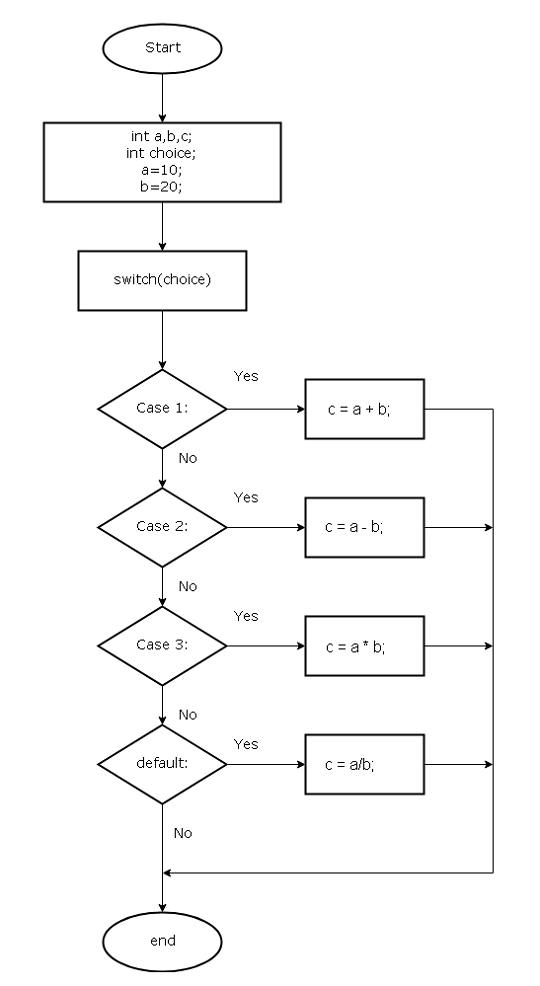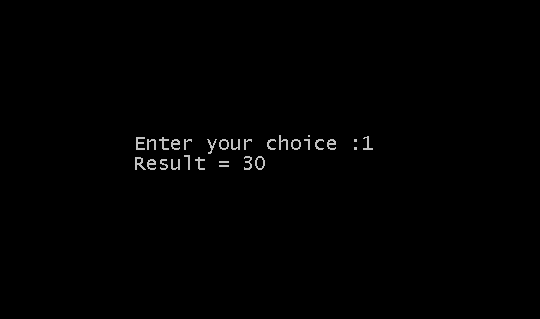## C Programming Tutorial – Flow Control – II

In the previous lesson, we learned about the flow control structure. We learned about the if-else structures and nested if structures. In this lesson, we will learn about another flow control feature – switch-case.

### If-else Construct

Consider a C program for calculator, the user is given a menu with options to choose from.

2. Subtract
3. Multiply
4. Divide

We can write a program using if-else construct for the above problem.

``````
#include <stdio.h>

int main()
{

int choice;

int a,b,c;

a = 10;

b = 20;

if (choice == 1)

c = a+b;

if (choice == 2)

c = a – b;

if (choice == 3)

c = a * b;

else if(choice == 4)

c = a/b;

else
{

printf(“Wrong input, Try again!”);

}

getch();

return 0;

}
``````

The user enters a number and it is matched with if-else blocks and whenever there is a match that block is executed.

The problem with these kinds of the construct is that when the menu is large, then the if-else construct will be difficult to manage. Also, there are many comparisons – each if a block is tested.

Switch-Case

The switch-case is suitable for menu driven C programs. Whenever the program is executed, a menu with a number of choices like the example below is presented to the user.

/* Calculator Application in C */

2. Subtract
3. Multiply
4. Divide

The switch block look like the following in program source code. The switch block accepts a number as user choice.

When the user enters a number next to an option and the Switch accepts the choice(number).

``````int choice;

Switch (choice)
{

}
``````

The switch () matches the user choice with a list of cases. Each of the cases has a number associated with them followed by a colon.

``````Case 2:

Statement1;

break;

Case 3:

Statement2;

Statement3;

break;
``````

When the user choice and the case number matches, the statements from the case is executed and terminated by a break; statement.

The entire switch-case block look like following

``````int choice;

scanf(“%d”,&choice);

Switch(choice)
{

Case 1:

Do something;

break;

Case 2:

Do something;

break;

default:

Do something;

break;

}
``````

Let’s create an example program using the Switch-Case. We shall write the Calculator program using the Switch-Case.

## Flowchart – C CalculatorFlowchart – Switch-Case

## Program Code

``````/* C Program for a Calculator using Switch-Case */

#include

int main()
{

int choice;

int a, b, c;

a = 10;

b = 20;

scanf (“%d”, &choice);

Switch (choice)
{

case 1:

c = a + b;

break;

case 2:

c = a - b;

break;

case 3:

c = a * b;

break;

case 4:

c = a / b;

break;

default:

printf (“Wrong choice, try again!”);

break;

}

printf (“Result = %d\n”, c);

getch();

return 0;

}
``````

Output

The output of the program is given below.Output-Switch-case

## Bibliography

Balagurusamy, E. 2000. Programming in ANSI C. Tata McGraw-Hill Education,.

Kanetkar, Yashavant. 20 November 2002. Let us C. Bpb Publications.